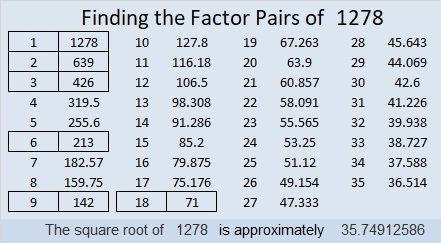# 1278 and Level 4

Try your hand at solving this level 4 puzzle. Your ability to do so might just surprise you!Print the puzzles or type the solution in this excel file: 12 factors 1271-1280

Since this is my 1278th post, I’ll write a little bit about that number:

• 1278 is a composite number.
• Prime factorization: 1278 = 2 × 3 × 3 × 71, which can be written 1278 = 2 × 3² × 71
• The exponents in the prime factorization are 1, 2, and 1. Adding one to each and multiplying we get (1 + 1)(2 + 1)(1 + 1) = 2 × 3 × 2 = 12. Therefore 1278 has exactly 12 factors.
• Factors of 1278: 1, 2, 3, 6, 9, 18, 71, 142, 213, 426, 639, 1278
• Factor pairs: 1278 = 1 × 1278, 2 × 639, 3 × 426, 6 × 213, 9 × 142, or 18 × 71,
• Taking the factor pair with the largest square number factor, we get √1278 = (√9)(√142) = 3√142 ≈ 35.749131278 and the four numbers immediately preceding it are the smallest consecutive numbers for which 4 of the 5 numbers each have exactly 12 factors.

1278 is the sum of eight consecutive prime numbers:
139 + 149 + 151 + 157 + 163 + 167 + 173 + 179 = 1278

This site uses Akismet to reduce spam. Learn how your comment data is processed.